American Journal of Civil Engineering
Volume 4, Issue 5, September 2016, Pages: 233-240

Dynamic Analysis of High Building with Cracks in Column Subjected to Earthquake Loading

Nguyen Thai Chung1, Hoang Hai2, Shin Sang Hee3

1Department of Solid Mechanics, Le Quy Don Technical University, Ha Noi, Viet Nam

2Ministry of Construction, State Authority for Construction Quality Inspection, Ha Noi, Viet Nam

3International Cooperation Division, Korea Institute of Civil Engineering and Building Technology, Goyang City, Korea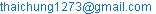(N. T. Chung)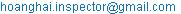(H. Hai)

Nguyen Thai Chung, Hoang Hai, Shin Sang Hee. Dynamic Analysis of High Building with Cracks in Column Subjected to Earthquake Loading. American Journal of Civil Engineering. Vol. 4, No. 5, 2016, pp. 233-240. doi: 10.11648/j.ajce.20160405.14

Received: June 25, 2016; Accepted: July 11, 2016; Published: August 2, 2016

Abstract: This paper is devoted to studying the dynamic response of a high building on an elastic foundation with cracks on the column of the building under earthquake loading using the finite element method (FEM). The authors used a 3D modeling frame - plate system and integration method developed by Newmark, along with construction calculation programs in the Matlab environment to analyze the problem. The results were reflected the effect of the cracks on the columns and other parameters.

Keywords: 3D Frame, Plate, Seismic Load

1. Introduction

2. Finite Element Simulation and Dominant Equations

A high building is modeled using the frame-plate composite system in the general coordinate system (X, Y, Z) under earthquake loading following X and Y directions as per Figure 1.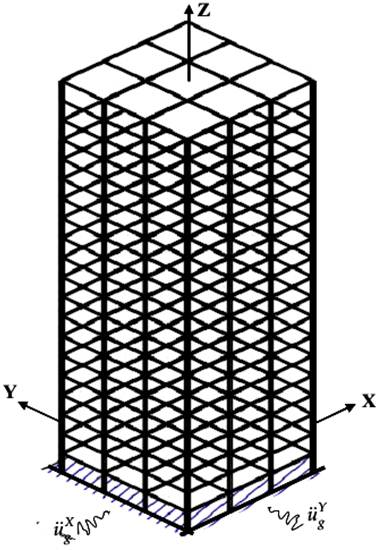Figure 1. Frame-plate composite system model to simulate high building.

Hypothesis: Materials have a structure of linear elasticity, and small deformation. Panel satisfies Reissner - Mindlin theoretical conditions. Only the horizontal acceleration of the foundation is considered. Construction work columns and foundation operate simultaneously.

2.1. Response Relation of Elements

2.1.1. Space Beam Element Describes the Frame

The beam element is shown in Fig. 2, and each node has 6 degrees of freedom, including ui, vi, wi,θxi, θyi, θzi.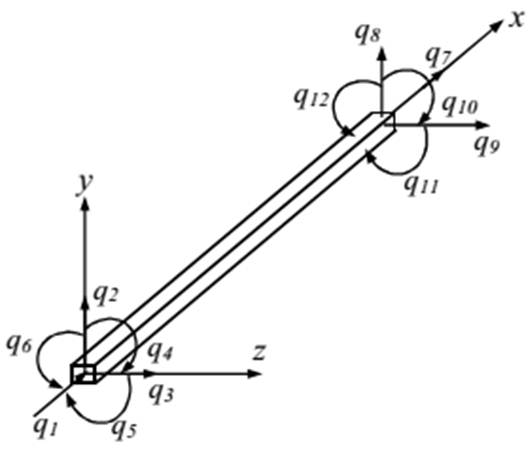Figure 2. Three dimension 2-node beam element model.

Displacement in any node of the bar with (x, y) coordinates is identified as follows , :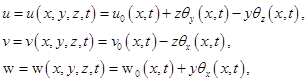(1)

where,

the subscript "0" represents axis x (y = 0, z = 0)

t represents time;

U, v and w are the displacements along x, y and z;

θx is the rotation of cross section about the longitudinal axis x; and

θx and θz denote rotations of the cross section about y and z axes.

The strain components: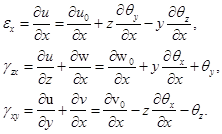(2)

Node displacement vector: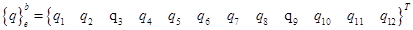(3)

Element stiffness matrix is set up from 4 types of component stiffness matrices: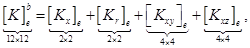(4)

where,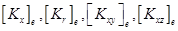are tension (compression) stiffness matrix, torsion stiffness matrix, bending stiffness matrix in the xy plane, and bending stiffness matrix in the xz plane, respectively.

In the (X, Y, Z) coordinate system, stiffness matrix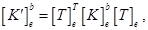(5)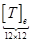is the coordinate axes transition matrix .

Similarly, element mass matrix is also established from 4 types of volume matrix: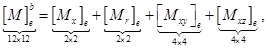(6)

In the (X, Y, Z) coordinate system(7)

node load vector of element in the natural coordinate system is: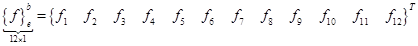(8)

In the (X, Y, Z) coordinate system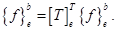(9)

The stiffness matrix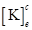of the cracked element can be written as: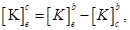(10)

where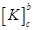is the reduction in the stiffness matrix due to the crack according to Peng et al. .

2.1.2. Plate Element Describes the Floors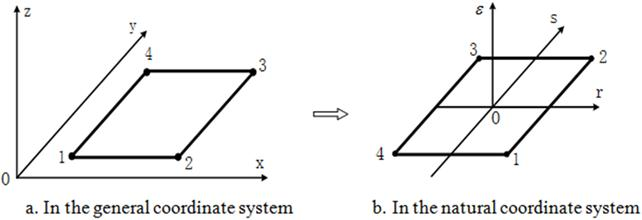Figure 3. Model of 4-node plate element and the coordinate system.

The displacement fields are written as , :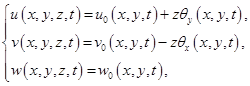(11)

where, u0, v0, w0 correspond to displacements of plate average, and θx, θy correspond to rotation angle of normal section of the plate plane in the survey node.

Deformation vector:(12)

where,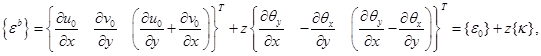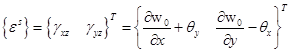(13)(14)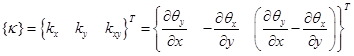(15)

Stress-deformation relation: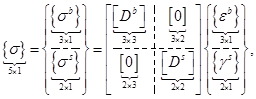(16)

Where,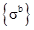is stress vector excluding shearing force,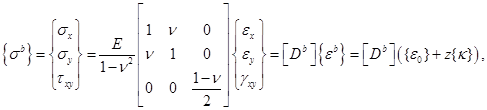(17)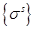is stress vector caused by horizontal deformation,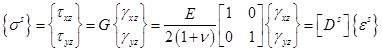(18)

E is Young’s modulus, and n is the Poisson coefficient of materials.

If using (17) and (18) relations, internal force vector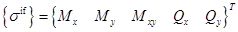is identified as follows: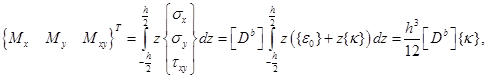(19)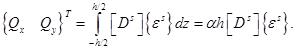(20)

Therefore: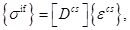(21)

where,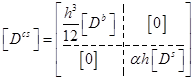,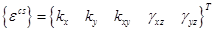- components of the bending deformation vector and shear deformation vector, and α = 5/6 is the coefficient including shear deformation.

Displacement components of any node of the element :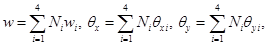(22)

where,

wi, θxi, θyi are displacements of w, θx, θy at node ith of the element, and written as

Ni is shape function of node i.

Therefore: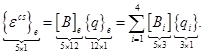(23)

where,

[B]e is matrix of strain - displacement relation,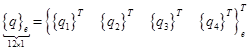is element node displacement vector, and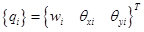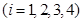.

Replacing (23) with (21) leads to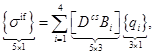(24)

where,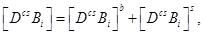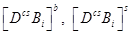- matrix for calculating bending moment and cutting force.

The total potential energy expression of the element is written as :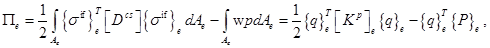(25)

where,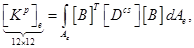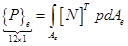are stiffness matrix and node load vector of the element,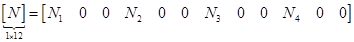Kinetic energy Te of the element :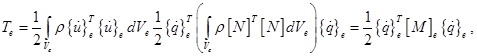(26)

where,

ρ is specific volume of material,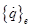is node velocity vector,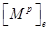is element mass matrix, and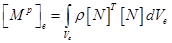(27)

2.2. Vibration Equation of the System

In the event of an earthquake, foundation acceleration causes acceleration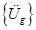for volume concentrated in nodes of the system, and represented as , :(28)

where,is soil acceleration vector,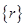is coefficient vector representing displacement in nodes due to horizontal displacement of soil by unit; vector structure includes elements 1 and 0 upon degrees-of-freedom of each node and number of foundation acceleration dimensions.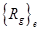is loading vector due to earthquake effecting nodes (force of inertial), caused by soil acceleration on node  convergence volume of the element.

We have :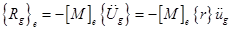(29)

Use of Hamilton principle for the element: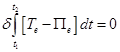(30)

where,

Te is kinetic energy and

Πe is potential energy of the element.

Then we have the equation of oscillation description of the element:(31)

After combining the matrices and element load vector to become general matrices, and load vector, we have the equation of vibration of the system as follows :(32)

where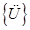,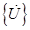, and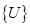are the acceleration, velocity, and displacement vectors of the system, respectively;

[M], [K], and {Rg}, {R0} are the mass, stiffness matrices, earthquake loading vector, and internal force vector, respectively: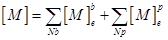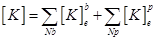(33)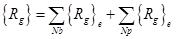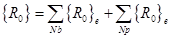(34)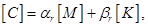(35)

where αr, βr are Rayleigh damping coefficients , .

After amplitude suppression, (32) becomes: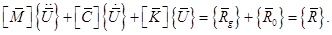(36)

This is a linear equation; the correct position of such equation depends on time. This equation is solved using the Newmark direct integral method and programming in the Matlab environment, including the earthquake loading vector updated after each time step.

3. Finite Element Algorithm

Step 2. Calculate initial parameters, give foundation acceleration chart function;

Step 3. Conduct integral cycle in each time step:

Update node load vector caused by earthquake;

Identify vectors of displacement, velocity, acceleration and internal force;

Step 4. Export results.

The calculation program was developed by the authors, and is called 3D_frame_earthquake.m.

4. Numerical Results and Discussion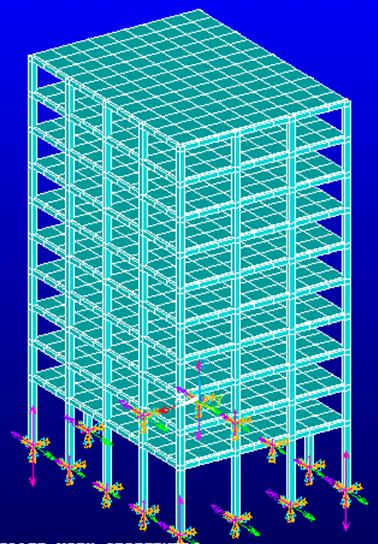Figure 4. Finite element modeling.

The 9–story building is calculated with the model of a frame-plate system, including 20 vertical columns, 90 beams and 10 floors. They are described to be flat slabs, the general story has a height of h0 = 5m. For the 9 remaining stories, each story has a height of hi = 3.5m, total height level of the building H = 6m + 9hi = 36.5m, width W = 4×6m = 24m, depth B = 3×6m = 18m. Cross section of columns is 0.55m×0.55m, beams are 0.3m×0.5m, and floors’ thickness is 0.15m. Columns and floors are made of reinforced concrete with properties of E = 3.64´1010N/m2, ν = 0.3, ρ = 2.76´103kg/m3. The middle column base of the building has a V- shaped crack with a depth of h0 = 0.1m. Finite element model of the building is shown in Fig. 4. The soil acceleration influencing 2 directions, including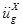,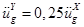is shown in Fig. 5.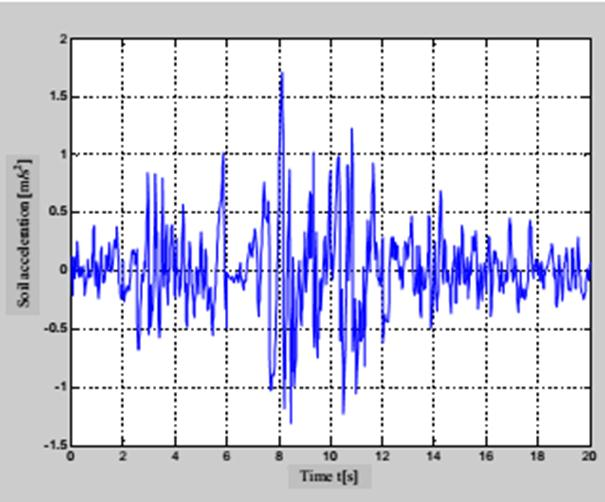Figure 5. Soil acceleration with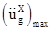=1,7085m/s2.

Results export: Central point of the 9th story’s floor (point A) and column base in the middle and front sides of the building (point B).

The displacement u at the point A and bending moment My at the point B are shown in Fig. 6, 7 following time.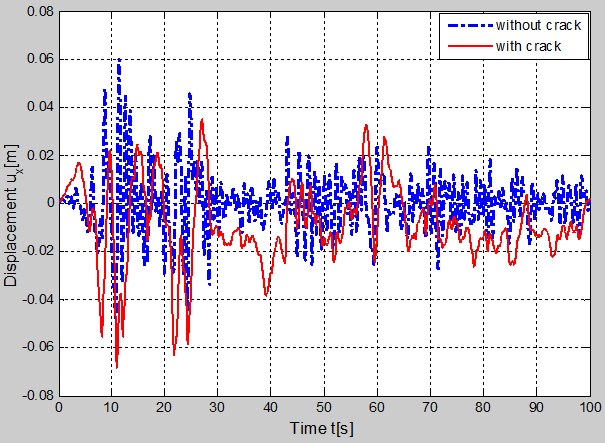Figure 6. Time history of u displacement.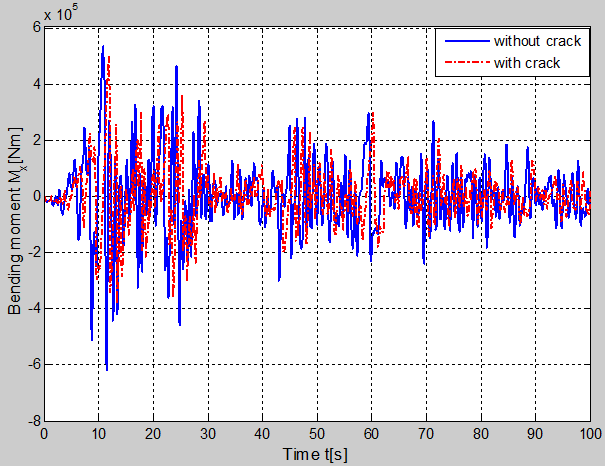Figure 7. Time history of bending moment.

The maximum displacement (umax) and bending moment (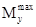) are shown in Table 1.

Table 1. The maximum of the displacement and bending moment (with crack and without crack).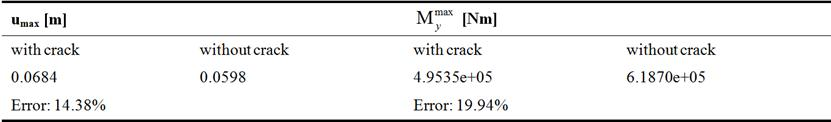The crack affects the dynamics of the building accordingly in the event of an increase in the cracks in the column, and the displacement of the building.

4.1. Influences of Damping

Survey is conducted in two scenarios, with damping and without damping. The results in terms of horizontal displacement and bending moment at points A and B are as shown in Figs. 8, 9 and Table 2.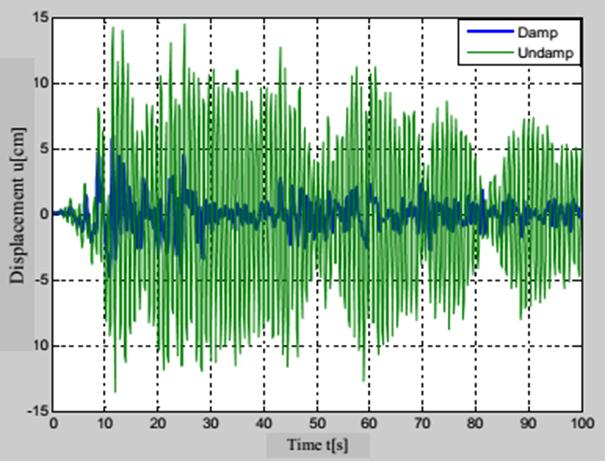Figure 8. Time history of u displacement.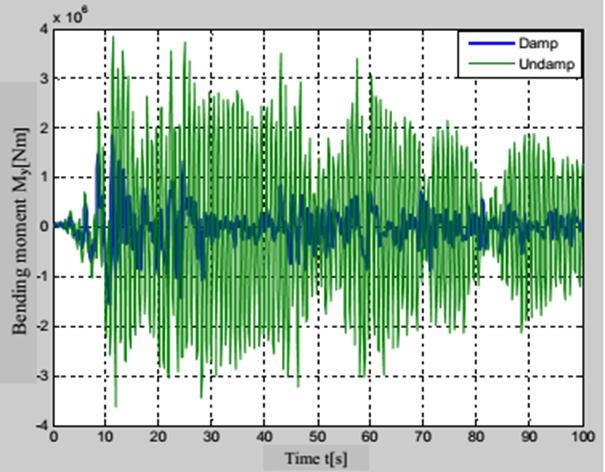Figure 9. Time history of bending moment.

Table 2. The maximum of displacement and bending moment (with damping and without damping).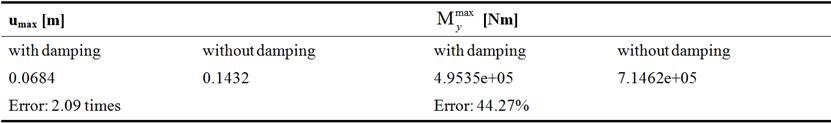The damping of the structures affects the dynamic of the building and reduces the displacement and internal force.

4.2. Influences of the Building Height

The relationship between the height of the building end the displacement of the floors of each story is shown in Fig. 10.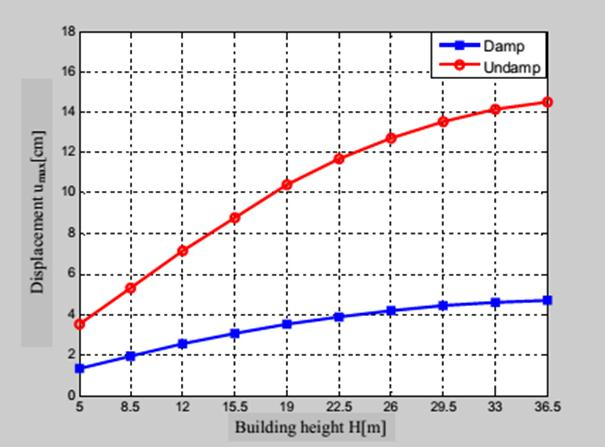Figure 10. Relationship between the height of the building and the displacement of floors.

Comment: Displacement of the building has a non-linear dependence on the height of the building; it increases quickly when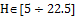m (2.98 times-with damping, 3.36 times-without damping) and slowly when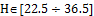m (1.28 times-with damping, 1.26 times-without damping). This shows that the solution of decreasing vibration in intermediary positions of the building is reasonable.

5. Discussion of Results

Our research arrived at several main results, including the development of an algorithm and dynamic analysis program for high buildings under earthquake loading, foundation acceleration following two orthogonal directions in the horizontal plane, and the use of a 3D modeling frame-plate system with cracks on the main column. The numerical analysis conducted in some Mathematics problems with changing parameters reflects the suitability of the calculation model; algorithm and calculation capacity of the program. The results contribute to the calculation, design and selection of reasonable solutions for new design, diagnosis of damages and reinforcement of modern high buildings.

References

1. Ming Ming Yao, (2010), Earthquake Wave-Soil-Structure Interaction Analysis of Tall Building, Doctoral Thesis, University of Victoria.
2. Mourad Nasser, (2012), Quality Assessment of Dynamic Soil-Structure Interaction Models Using Energy Measures, Doctoral Thesis, Bauhaus-University at Weimar Faculty of Civil Engineering.
3. Sushma Pulikanti (2013), Affect of soil-foundation-structure interaction on seismic behaviour of pile supported frame building, Doctoral Thesis, International Institute of Information Technology - Hyderabad.
4. Aslan Sadeghi Hokmabadi, (2014), Effect of Dynamic Soil-Pile-Structure Interaction on Seismic Response of Mid-Rise Moment Resisting Frames, Doctoral Thesis, Faculty of Engineering and Information Technology University of Technology Sydney (UTS).
5. Ahmed Abdelrahem Farghaly and Ali Mohamed Abdallah (2014), Evaluation of Seismic Retrofitting Techniques Used in Old Reinforced Concrete Building, IOSR Journal of Engineering (IOSRJEN), ISSN (e): 2250-3021, ISSN (p): 2278-8719, Vol. 04, Issue 06 (June. 2014), pp. 14-22.
6. D. K. Jain and M. S. Hora, (2014), Interaction analysis of space frame-shear wall-soil system to investigate foundation forces under seismic loading, Department of Aerospace Engineering, Korea Advanced Institute of Science and Technology.
7. J. Sandhya Rani, P. Srinivas Reddy, M. Koti Reddy (2015), Time history analysis of building structures with water tank as passive tuned mass damper, International Journal of Research in Engineering and Technology eISSN: 2319-1163, Pissn: 2321-7308, pp. 84-90.
8. Anil K. Chopra (2004), Dynamics of structures theory and applications to earthquake engineering (Second edition), Pearson education Asia limited and Tsinghua university press.
9. Bathe K. J and Wilson E. L (1978), Numerical Method in Finite Method Analyis Prentice , Hall of India Private Limited, New Delhi.
10. David J. Dowrick (1988), Earthquake resistant design for engineers and architects, Singapore, Chichester, New York, Brisbane, Toronto.
11. Stanislav Stoykov (2012), Nonlinear vibrations of 3D beams, Doctor of Philosophy, University of Porto.
12. Wolf J. P (1988), Dynamic Soil-Structure Interaction Analysis in Time domain, Prentice-Hall Inc. Englewood Cliffs, N. J. 07632.
13. Reddy J. N (2004), Mechanics of Laminated Composite Plates and Shells: Theory and Analysis, CRC Press.
14. Peng, Z. K., Lang, Z. Q., Billings, S. A. (2007), Crack detection using nonlinear output frequency response functions, Journal of Sound and Vibration, 301. Pp. 777-788.
15. Nguyen Thai Chung, Hoang Hai and Nguyen Quang Minh (2015), Dynamic analysis of tall building in the 3D frame-plate under seismic load, Journal of Construction, Vietnam, pp. 65-69.

 Contents 1. 2. 2.1. 2.2. 3. 4. 4.1. 4.2. 5.
Article ToolsAbstractPDF(2566K)